# MCQs on Power Systems

##### Page 11 of 67. Go to page 1 2 3 4 5 6 7 8 9 10 11 12 13 14 15 16 17 18 19 20 21 22 23 24 25 26 27 28 29 30 31 32 33 34 35 36 37 38 39 40 41 42 43 44 45 46 47 48 49 50 51 52 53 54 55 56 57 58 59 60 61 62 63 64 65 66 67
01․ Condition for maximum voltage regulation
tanφ = X/R
tanφ = R/X
cosφ = X/R
cosφ = R/X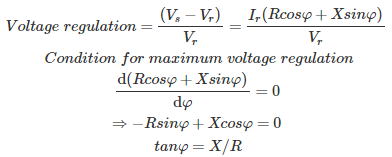02․ Condition for zero voltage regulation is
tanφ = X/R
tanφ = R/X
cosφ = X/R
cosφ = R/X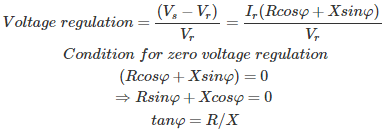03․ Zero voltage regulation is possible only for
lagging power factor
unity power factor
all of the above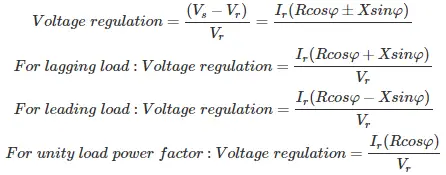Therefore, zero voltage regulation is possible only for leading power factor.

04․ A 1-phase transmission line has an impedance (3+4j) Ω . Find the power factor of the load for achieving maximum and zero voltage regulation?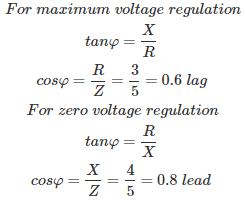05․ A transmission line has an impedance of (2+j6) Ω has voltage regulation of 10% at a load power factor of 0.8 lag. Find the voltage regulation for a load of 0.6 lead
6.92%
10%
-6.92%
-10%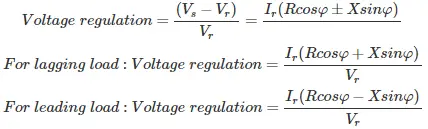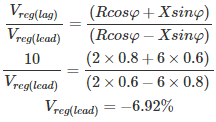06․ A transmission line has 50 km operating at frequency of 500 Hz. Find the type of the line?
short transmission line
long transmission line
medium transmission line
any of the above

Classification of transmission lines: Short transmission line = Length(L) < 80 km and product of length(L) and frequency(f) L.f< 4000 Medium transmission line = 80 km ≤ L ≤ 200 Km and 4000 ≤ L.f ≤ 10000 Long transmission line = L > 200 km and L×f >10000 Given that L = 50 km f = 500 Hz L.f = 50×500 = 25000 Therefore given line is a long transmission line.

07․ A transmission line has 200 km operating at frequency of 10 Hz Hz. Find the type of the line
short transmission line
long transmission line
medium transmission line
any of the above

Classification of transmission lines: Short transmission line = Length(L) < 80 km and product of length(L) and frequency(f) L.f< 4000 Medium transmission line = 80 km ≤ L ≤ 200 Km and 4000 ≤ L.f ≤ 10000 Long transmission line = L > 200 km and L×f >10000 Given that L = 200 km f = 10 Hz L.f = 200×10 = 2000 Therefore given line is a short transmission line.

08․ In which of the following transmission lines capacitance effect is negligible?
long transmission lines
shoart transmission lines
medium transmission lines
both 1 and 3

Short transmission line = Length(L) < 80 km and product of length(L) and frequency(f) L.f < 4000 For short transmission line charging current and leakage currents are very small. So that capacitance effect and conductance are negligible only for short transmission lines.

09․ Communications lines are treated as
short transmission lines
long transmission lines
medium transmission lines
any of the above

Communication lines are operating at the MHz range. So that communication line is represented as long transmission line equivalent circuit. In which all the transmission line parameters are i.e., R,L,C and G are considered. Classification of transmission lines: Short transmission line = Length(L) < 80 km and product of length(L) and frequency(f) L.f < 4000 Medium transmission line = 80 km ≤ L ≤ 200 Km and 4000 ≤ L.f ≤ 10000 Long transmission line = L > 200 km and L.f >10000

10․ If the rated receiving end voltage is 33 kV, then what are the limits for safe operation of equipments?
33 to 50 kV
25 to 35 kV
31.35 to 34.65 kV
any voltage above 33 kV

Due to transmission line losses and other losses, we can not get the rated voltage to the equipments. So that equipments are designed to operate at ± 5% or ± 6%. Therefore, the limits of safe operation of equipments are 31.35 to 34.65 kV. Beyond this limits equipment may damage or not operate.

<<<910111213>>>